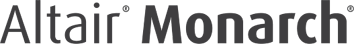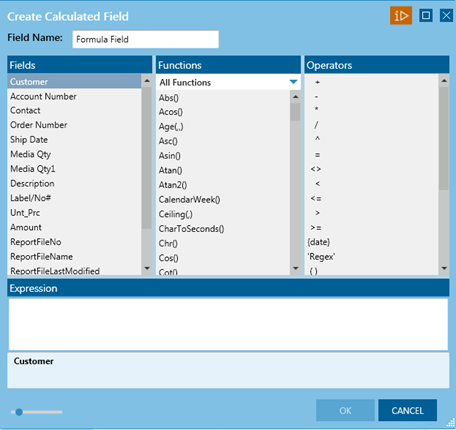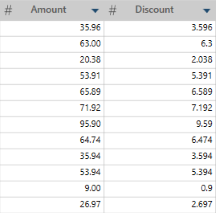# Creating Calculated Fields

### Description

Creates a new column based on a calculation.

### Steps

1. Right+Click on a column and select Create Calculated Field... from the context menu. The Create Calculated Field dialog displays:1. Enter the new column name.

2. Define the expression for calculating the new field value:

• Select fields, operators, functions, and operators from the lists above the expression builder.

• Refer to Functions Reference for a list and description of all functions you can use within an expression.

### exampleS

In this example, the first column (Amount) is the original column. Discount is a calculated field that shows the 10% discount value. It uses the following simple formula: Amount * .10:Other expressions can look like this:

• If(Customer="Ace Resellers",Unit_price*.55, Unit_price*.65)

• FiscalWeek([Ship Date])

• Replace(Contact,"Smith","Smith-Jones")